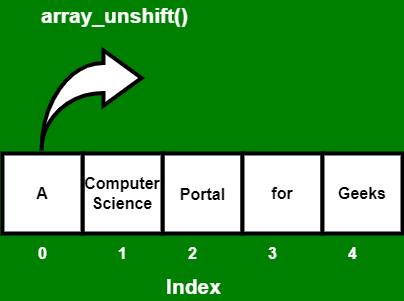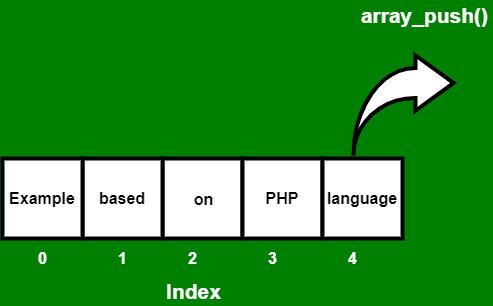# How to insert a new element in an array ?

• Difficulty Level : Basic
• Last Updated : 22 Nov, 2021

In PHP, an array is a type of data structure that allows us to store similar type of data under a single variable. The array is helpful to create a list of elements of similar types, which can be accessed using their index or key.

We can insert an element or item in an array using two functions which is:

• array_unshift() function
• array_push() function

Using array_unshift() function This function is used to add one or more than one element at the beginning of the array. and these elements get inserted at the beginning of the array. because of the inserted elements or items in the array, the length of the array also gets increased by the number of elements inserted or added in the array. After inserting the elements, we can use the print_r() function to print the array information.

Below are examples of how to do it: Let’s see how to insert an element at the beginning of the array using the array_unshift() function.

```Input:  names[] = array("portal", "for", "geeks"), size = 3, capacity = 3
Output: names[] = array("_", "_", "portal", "for", "geeks"), size = 5, capacity = 5```

Example 1: In this example, we use an indexed array to insert elements at the beginning of the array using the array_unshift() function.## PHP

 ``

Output

```Array
(
 => A
 => Computer science
 => portal
 => for
 => geeks
)```

Example 2: In this example, we use an associative array to insert elements at the beginning of the array using the array_unshift() function

## PHP

 ` ``"CSS"``, ` `      ``"language2"` `=> ``"PHP"``, ` `      ``"language3"` `=> ``"MySQL"` `);`   `array_unshift``(``\$array``, ``"HTML"``);` `print_r(``\$array``);`   `?>`

Output

```Array
(
 => HTML
[language1] => CSS
[language2] => PHP
[language3] => MySQL
)```

Using array_push() functionThis function is used to push new elements into an array. We can push one or more than one element into the array and these elements get inserted to the end of the array and because of the pushed elements into the array, the length of the array also gets incremented by the number of elements pushed into the array. After inserting the elements, we use the print_r() function to print the array information.

Below are examples of how to do it: Let’s see how to insert an element at the end of the array using the array_push() function.

```Input:  arr[] = array("Example", "based", "on"), size = 5, capacity = 5
Output: arr[] = array("Example", "based", "on", "_", "_"), size = 5, capacity = 5```

Example 3: In this example, we use an indexed array to insert elements at the end of the array using the array_push() function.## PHP

 ``

Output

```Array
(
 => Example
 => based
 => on
 => PHP
 => language
)```

Example 4: If we want to add a single element at the end of an array.

## PHP

 ``

Output

```Array
(
 => Technical
 => Content
 => Writer
 => at GFG
)```

Using array_merge() functionThe array_merge() function is used to merge two or more arrays into a single array. This function is used to merge the elements or values of two or more arrays together into a single array. The merging occurs in such a manner that the values of one array are appended at the end of the previous array.

Example 5: The array_merge() function merges element of one or more than one arrays into one array.

## PHP

 ``

Output

```Array
(
 => GFG
 => stands
 => for
 => Geeks
 => for
 => Geeks
)```

My Personal Notes arrow_drop_up
Recommended Articles
Page :